### Testing the Global Null Hypothesis

The following statistics can be used to test the global null hypothesis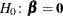. Under mild assumptions, each statistic has an asymptotic chi-square distribution with
degrees of freedom given the null hypothesis. The valueis the dimension of. For clustered data, the likelihood ratio test, the score test, and the Wald test assume independence of observations within a cluster, while the robust Wald test and the robust score test do not need such an assumption.

#### Likelihood Ratio Test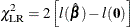#### Score Test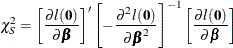#### Wald’s Test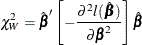#### Robust Score Test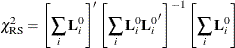where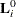is the score residual of the ith subject at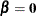; that is,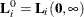, where the score process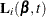is defined in the section Residuals.

#### Robust Wald’s Test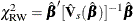where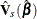is the sandwich variance estimate (see the section Robust Sandwich Variance Estimate for details).